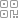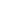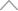6 0 7 3 9 3 1

​LeetCode刷题实战497：非重叠矩形中的随机点

https://leetcode-cn.com/problems/random-point-in-non-overlapping-rectangles/• 整数点是具有整数坐标的点。

• 矩形周边上的点包含在矩形覆盖的空间中。

• 第 i 个矩形 rects [i] = [x1，y1，x2，y2]，其中 [x1，y1] 是左下角的整数坐标，[x2，y2] 是右上角的整数坐标。

• 每个矩形的长度和宽度不超过 2000。

• 1 <= rects.length <= 100

• pick 以整数坐标数组 [p_x, p_y] 的形式返回一个点。

• pick 最多被调用10000次 。

示例

["Solution","pick","pick","pick"]

[[[[1,1,5,5]]],[],[],[]]

[null,[4,1],[4,1],[3,3]]

["Solution","pick","pick","pick","pick","pick"]

[[[[-2,-2,-1,-1],[1,0,3,0]]],[],[],[],[],[]]

[null,[-1,-2],[2,0],[-2,-1],[3,0],[-2,-2]]

解题

https://blog.csdn.net/weixin_44171872/article/details/111083657

（1）两次随机过程，先使用随机找到点所在的矩形，再使用随机确定该矩形内的一个位置；

（2）随机确定矩形的过程，可以通过面积来进行映射，计算出矩形的总的面积，然后将随机数对该总面积取余，将余数映射到某个矩形；

（3）找到该矩形后，可以对使用随机数对该矩形的高和宽分别取余映射，获得两个值，将这两个值加上左下角，即为坐标位置；

class Solution {

public:

vector<vector<int>> _rects;//统计各个矩形

vector<int> _area;//获得各个矩形对应的面积

long _sum_area;//总的矩形面积

Solution(vector<vector<int>>& rects):_rects(rects) {

_area.reserve(_rects.size());

_sum_area=0;

for(vector<int>& rect:_rects){

_area.push_back((rect-rect+1)*(rect-rect+1));//获得各个矩形的面积

_sum_area+=_area.back();

}

}

vector<int> pick() {

long sum_area=rand()%_sum_area+1;//将随机数映射到矩形中

int index=0;

//找出是第几个矩形

while(_area[index]<sum_area){

sum_area-=_area[index];

++index;

}

vector<int>& cur_rect=_rects[index];

//对该矩形的高和宽进行区域，映射到矩形内的点

int x=rand()%(cur_rect-cur_rect+1);

int y=rand()%(cur_rect-cur_rect+1);

return {cur_rect+x,cur_rect+y};//加上矩形的左下角，即为坐标

}

};

新知精选

IT研发知识▪优秀创作者

优选课程 新知学院

•扫码下载商业新知APP~

•回到顶部